# Module 3: System Partitioning

cancel
Showing results for
Did you mean:

## Module 3: System Partitioning

7,025 ViewsNXP Employee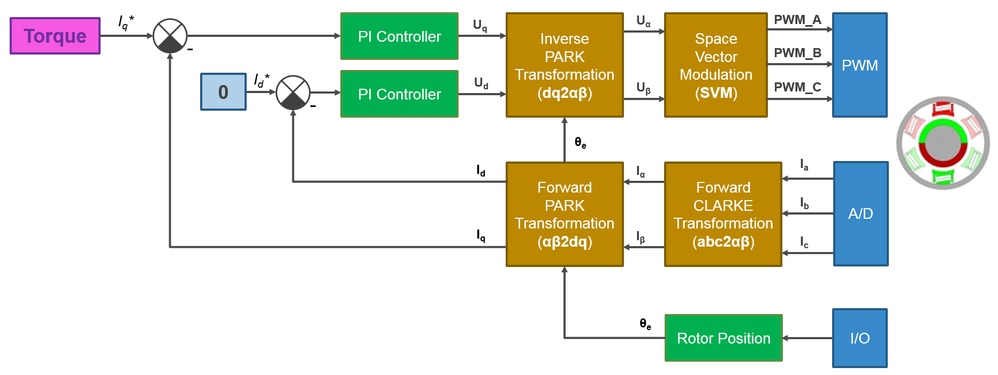INTRODUCTION

In this module of the, the focus is on the Field Oriented Control (FOC) specific steps required to build a digital control system used to control the speed and position of PMSM's rotor.

The goal of this article is to explain step-by-step all the aspects involved in FOC transformations starting from current measurements, space vector generation and transformation from a stationary reference frame into a rotating synchronous one, flux and torque control techniques via PI regulators that commands the appropriate voltages to align the stator magnetic flux into the correct position to have the PMSM controlled into the most efficient way.

Video training: How To Build a Field Oriented Control System for PMSM Using S32K144EVB

(view in My Videos)

Please watch this video before going further. The rest of the article is a summary of the video.

`         `

CURRENTS MEASUREMENT

First, as general assumption we will consider that the motor windings forms an isolated 3-phase symmetric system. This implies that the phase currents can only flow thru the motor stator coils – the windings being connected in start or delta configuration and there is no current path thru the PMSM stator frame.

Typically, on most of the PMSM especially the small and medium size, there are 3 wires that connects the motor internal windings with the external power sources. Since this is a isolated system, it means that we have to measure only 2 phase currents and then apply the Kirkoff’s current law to compute the 3rd phase current. Using 2 shunt resistors we can measure the voltage drop across these and then convert the shunt voltages with a current values with the help of a A/D converter as shown in Fig. 1.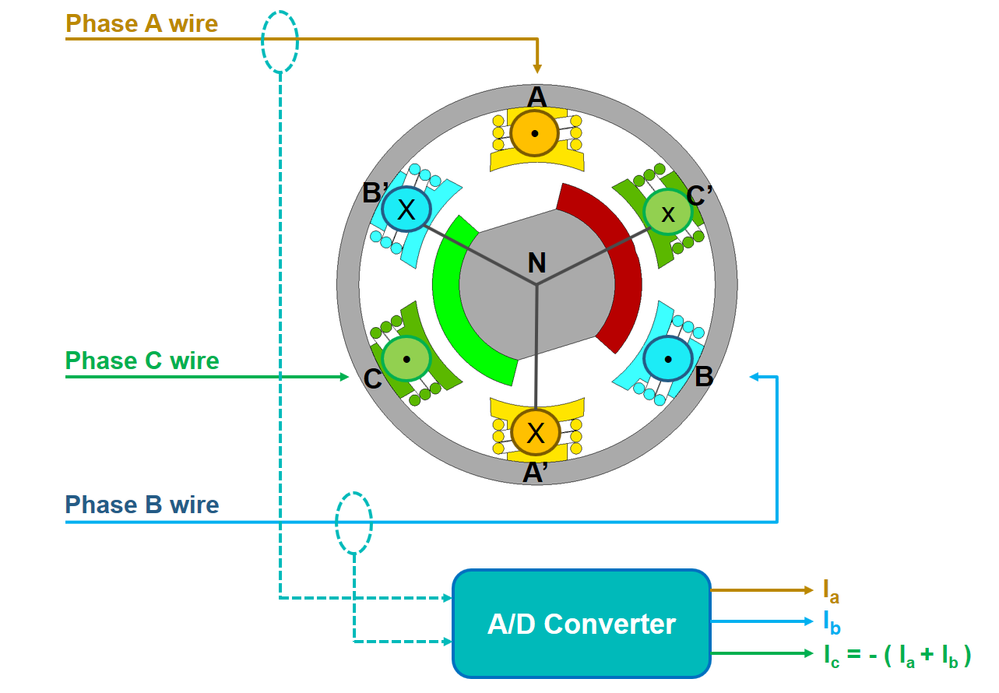Fig. 1: 3-phase currents measurement with 2 shunt resistors and A/D Converter

Further on, we can represent each measured current on the 3-phase stationary reference frame. Assuming some particular values for the phase currents we can easily compute the resulting stator current vector Is that will control the stator magnetic flux by adding these 3 phase current vectors using the parallelogram low of vectors as shown in Fig. 2.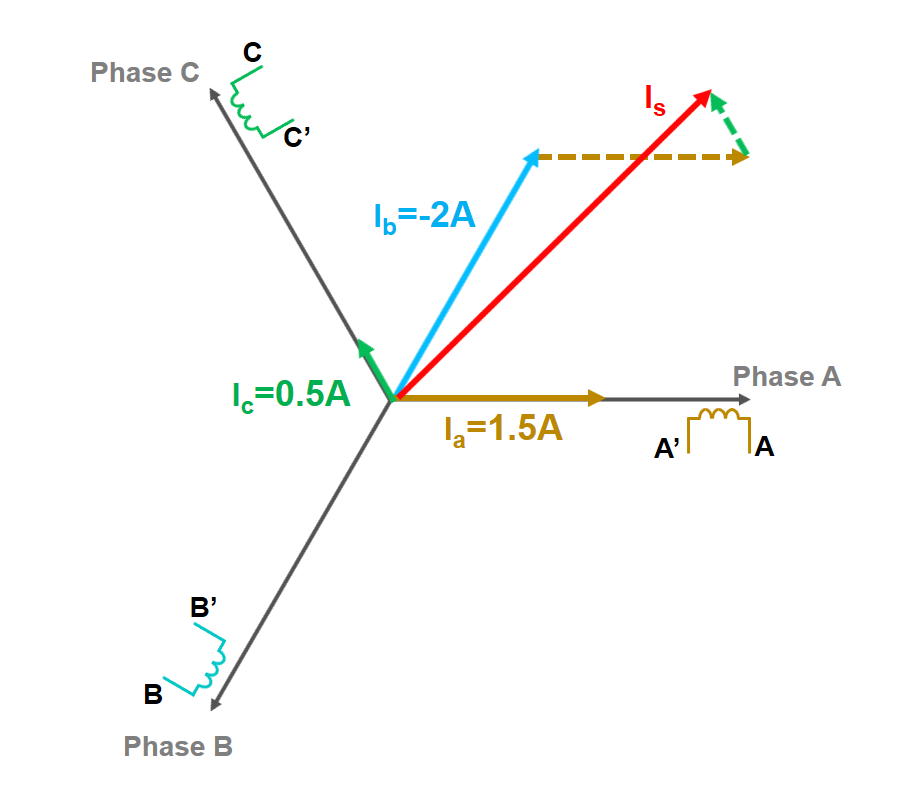Fig. 2: Stator current vector Is representation in the 3-phase fixed reference frame

CURRENT VECTOR REGULATION

Once we have the measured stator currents and we form the vector representation of those phase current in the fixed reference frame, we need to check if the resulting stator current vector has the correct magnitude and orientation.

In Module 2: PMSM and FOC Theory we have discussed that the magnetic flux produced by the stator current vector should be oriented at 90 electrical degrees compared to the rotor magnetic flux vector in order to maximize the torque produced by the PMSM per unit of amp injected in the stator coils.

Therefore, the main question: how is the rotor magnetic flux oriented ?

Assuming we have a way to measure or to estimate the PMSM's rotor position then it is relatively simple to find out the stator current vector orientation since we know the angle between the motor magnetic phase A and rotor flux axis.

Lets us assume that the rotor is in the position shown on Fig. 3 on left hand side. If that is the case then perhaps the Is orientation is correct – 90 degrees relative to rotor flux axis.

But how about the rotor being in the position indicated in Fig. 3 on the right hand side? In this case we need to re-orient the stator current vector to make sure the motor develops all the time the maximum torque per amps.

Now, you can easily understand the role of regulating current vector in Field Oriented Control. The main goal is to be able to compute the correct position of the reference stator current vector and drive the actual measured current vector into that position – ultimately we want to minimize the error vector shown in Fig. 3 with magenta and keep it to zero.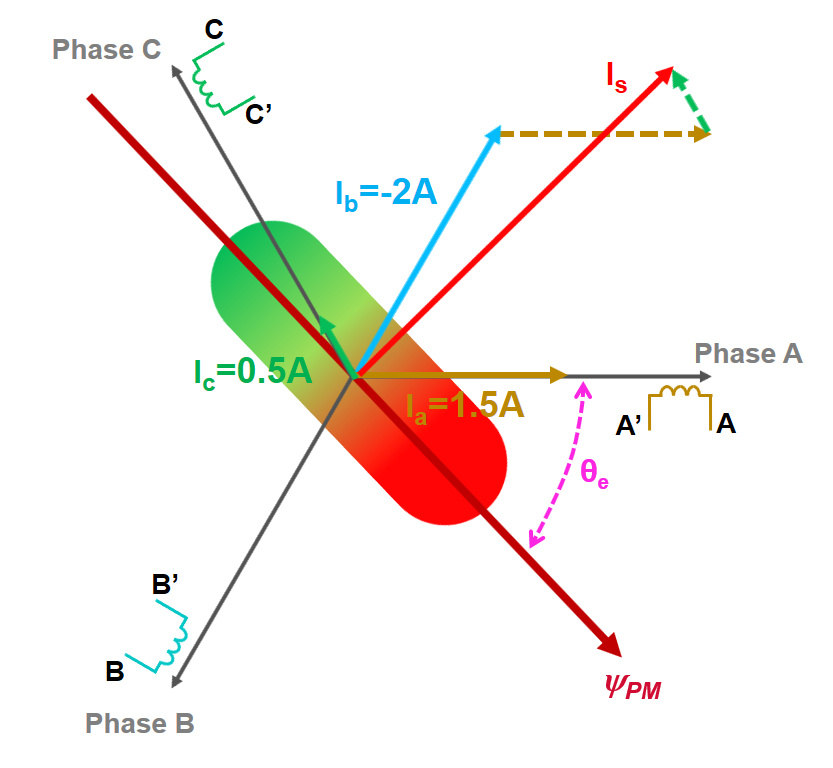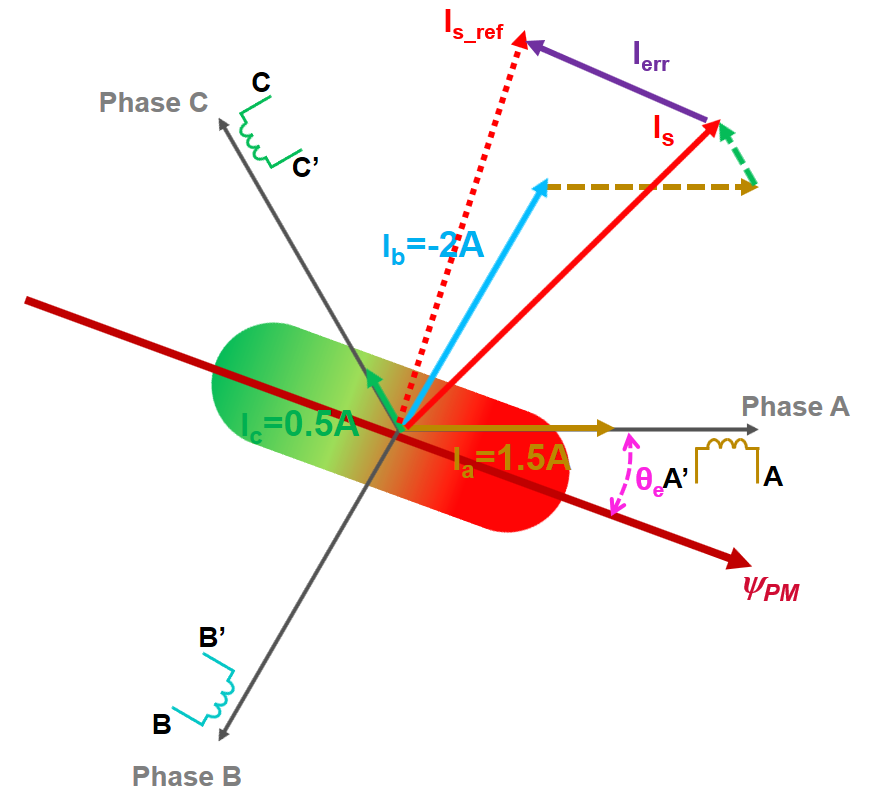Fig. 3: Stator current vector possible aligning options depending on the PMSM rotor flux axis actual position

CURRENT VECTOR CORRECTION

In order to keep the stator magnetic flux all the time at 90 degrees relative to the rotor magnetic flux produced by the magnets to maximize the motor efficiency, all we have to do, is to force into the motor windings a new set of phase currents that will create a new stator current vector aligned with the reference vector we wish to target as in Fig. 4 - right hand side.

In this case, a possible solution would be to increase the current in phase C, decrease the one in phase A and keep unchanged the current flowing in phase B – as depicted in Fig. 4.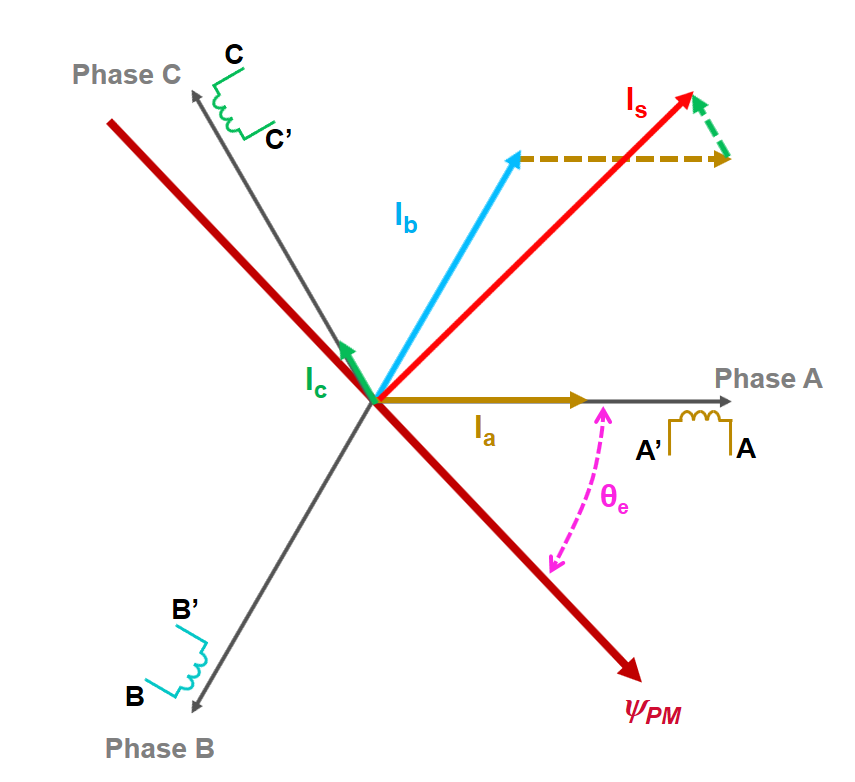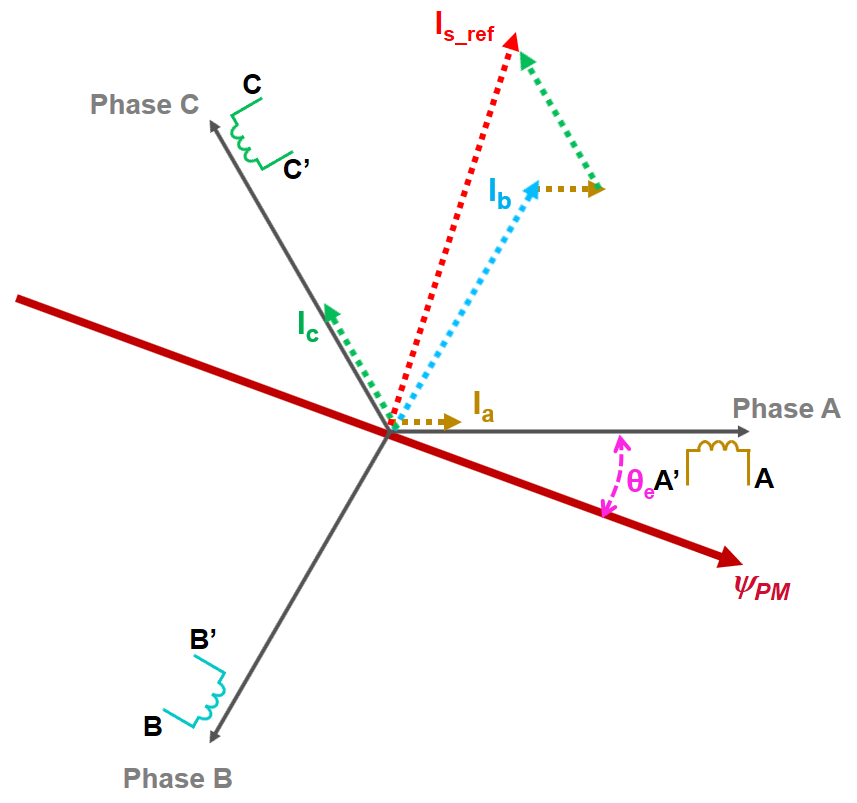Fig. 4: Generate a new set of phase current to correct the position of the stator vector current - before (left) and after (right

In general that is easier said than done!!!

All these vectors shown until now are a representation in fixed reference frame of some values that are time variant. We could build a control system capable of tracking and regulating sinusoidal quantities and most likely it would behave relatively ok-ish for most of the use-cases.  But designing such controllers is a tedious task and typically such control systems pose a lot of challenges in terms of frequency response and phase shift of the system. Usually such control systems will fail at high speed changing rate.

Anyhow – it will be waste a time even trying such approach since now we know there is a better way.

Field Oriented Control helps us with the use of trigonometry to solve this problem in a elegant way. Instead of trying to build and tune controllers capable of dealing with AC quantities, we can transform the values that we are measuring from a stationary to a rotating reference system. By using such transformations we can get rid of sinusoidal variation of the signals and deal only with continuous signals.

That is the beauty of FOC. Using simple equations we can move from one complex system into a more convenient one where the regulators can operate on DC quantities. We do all the control computation in this domain and then using same transformations but time in reverse we translate that information in something useful can be used to control the actual motor.

CLARKE AND PARK TRANSFORMATIONS

First step is to simplify the equations by moving from a 3-phase fixed system into an orthogonal fixed system. This is allowed since all the transformations happens in the same two-dimensional plane. This orthogonal system is called alpha-beta and typically the alpha axis is aligned with 3-phase system phase A.

The same vector Is can be easily represent in alpha-beta reference system as simple projections on the 2 orthogonal axes  instead of 3 ones phased out at 120 degrees apart as shown in Fig. 5.

This transformation is called forward Clarke and is basically a group of 3 multiplications and one addition operations.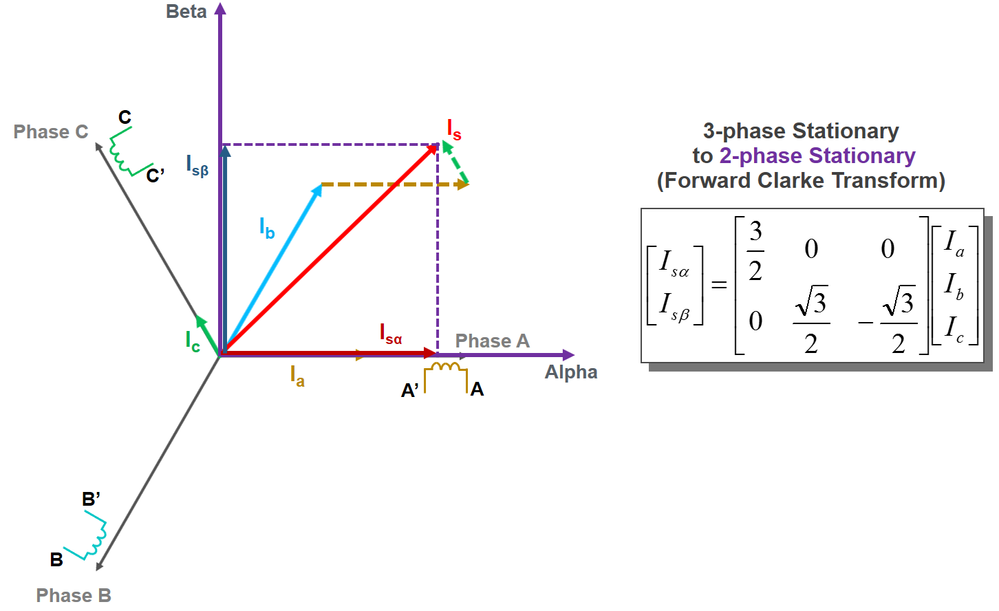Fig. 5: Clarke Transformation simplifies the model by reducing the number of variables we need to deal with

Now imagine (see Fig. 6) we create a new two axis frame, however this one is not being stationary but instead we will let rotate this two axis frame in the same direction and speed of the rotating current vector Is.

In the DQ rotating frame the space vector Is is described by a constant value of Id and Iq components which means that the position and amplitude of the space vector Is can be fully controlled by two DC values.

And this is how the Park transformation simplifies the control structure further down. Now there is no need to cope with AC quantities any more, which are difficult to control by conventional methods in a wider frequency range.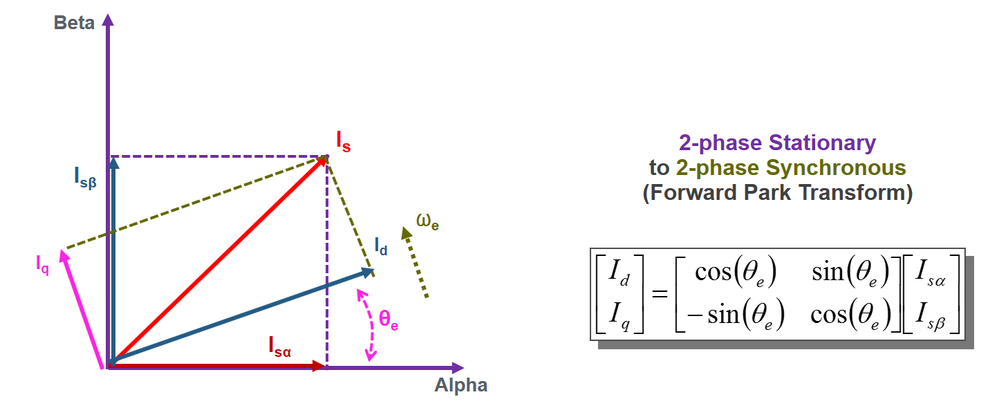Fig. 6: ParkTransformation simplifies the model by transforming AC -> DC quantities

APPLICATION MAPPING ON S32K PRODUCT FAMILY

It is now time to map the algorithm we have just discussed on top the the existing hardware we have presented in Module 1: Environment Setup .

The block diagram is shown in Fig. 7. The top box is showing the power stage that is available via Motor GD development kit and the bottom box shows the functionalities implemented in the S32K144 evaluation board.

The light blue blocks are implemented in hardware on the S32K144 while the yellow blocks are implemented in software with the help of Model-Based Design Toolbox for S32K1xx Automotive MCU v3.0.0 directly from Matlab/Simulink.

We'll have two control loops in this implementation:

• The inner loop or fast control loop represented here by the FOC block, takes as inputs the currents measured via dedicated ADC channels, the rotor position and the commanded torque value. Once the corrective actions are computed, the FlexPWM duty cycle values will be updated and sent to the pre-driver to be applied as a voltage on motor phase. The FlexPWM is also configured to trigger a hardware interrupt to the programmable delay blocks (PDB) that controls the ADC conversions at specific time intervals.
• In the outer speed control loop a given target velocity, being sent over FreeMaster for example, is compared to the actual measured speed and regulated in the speed PI controller which modifies torque reference.

Via UART or CAN the FreeMaster is integrated in our application  and communicates the status of the control loop variables. Various other HW and SW modules are used to configure and protect the power stage or to inform the user about potential faults in the system.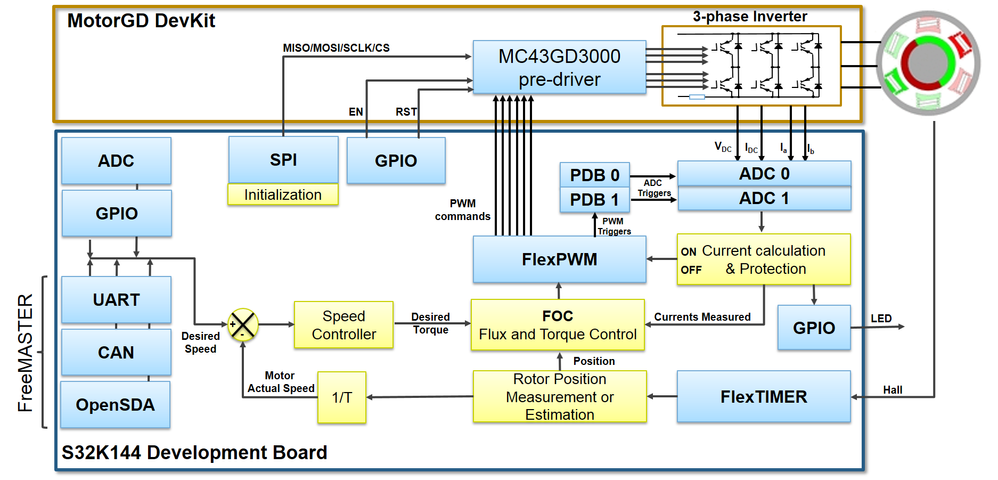Fig. 7: Application mapping of software and hardware used in to develop FOC over the S32K144EVB and MotorGD DevKit

We hope you find this information useful. Feel free to LIKE this article and comment below.

0 Replies